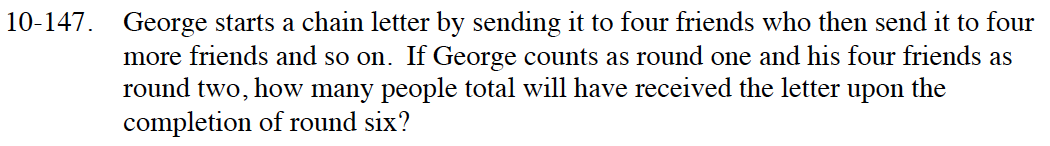Home > CCA2 > Chapter 10 > Lesson 10.3.1 > Problem10-147

10-147.

George starts a chain letter by sending it to four friends who then send it to four more friends and so on. If George counts as round one and his four friends as round two, how many total people will have received the letter upon the completion of round six? Homework Help ✎What are the first three terms in the sequence? Find the pattern.

t(1) = 1
t(2) = 4
t(3) = 16
so, t(n) = 4n − 1

Find t(6). What is the common ratio?

Now that you know r = 4, t(k) = 1024, and t(1) = 1, you can find the total number of people that will receive the letter by using the formula:

$S(k)=\frac{r\cdot t(k)-t(1)}{r-1}$

1365 people